Geometry

Area of a circle calculator

Area of a circle calculator - calculating area of a circle online. Area of a circle - is a numerical characteristic that characterizes the size of a plane bounded by a circle line. Area of a circle can be calculated using the number pi and the radius of the circle, or using other known input data.

1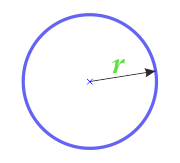... preparation ...

2

Circle area through diameter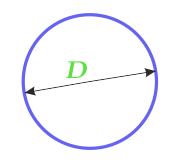D - diameter

... preparation ...

3

Area of a circle along the circumference- circumference
... preparation ...

4

Area of a circle through a square inscribed in a circle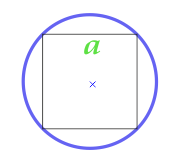a - side

... preparation ...

5

Area of the circle inscribed in a square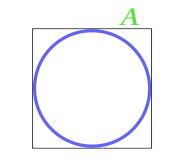A - side

... preparation ...

6

Area of the circle described around an arbitrary triangle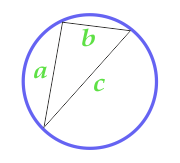This formula is applicable only if a circle can be described around a triangle, that is, all three vertices of the triangle must lie on the line of the circle. The triangle in this case can be any.

To calculate the area of a circle, first calculate the semi-perimeter of a triangle

a - side
b - side
c - side
... preparation ...

7

Area of a circle described near an equilateral triangle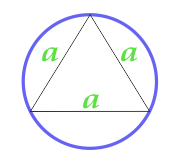a - side

... preparation ...

8

Area of a circle described around an equilateral triangle, calculated from the height of the triangle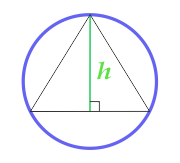h - height

... preparation ...

9

Area of a circle about an isosceles triangle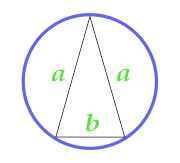a - side
b - base

... preparation ...

10

Area of the circle described about a right triangle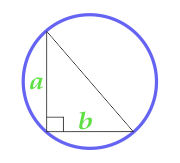a - side
b - side

... preparation ...

11

Area of a circle inscribed in an isosceles triangle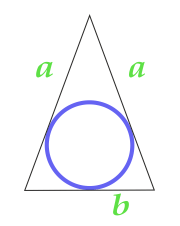a - side
b - base

... preparation ...

12

Area of a circle inscribed in an isosceles triangle, calculated on the sides of the triangle and the angle between them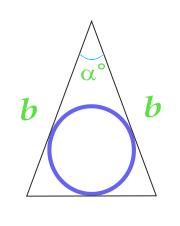b - side
α - angle between parties

... preparation ...

13

Area of a circle inscribed in a right triangle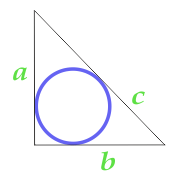a - side
b - side
c - side

... preparation ...

14

Area of a circle inscribed in a right triangle, calculated by the side and angle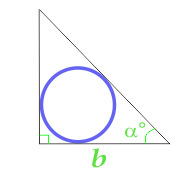b - side
α - angle at base

... preparation ...

15

Area of a circle inscribed in an equilateral triangle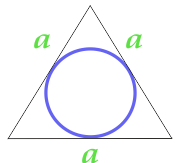a - side

... preparation ...

16

Area of a circle inscribed in an isosceles trapezium, calculated from the base of the trapezium and the angle at the base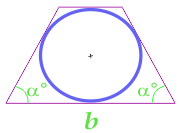b - side
α - angle at base

... preparation ...

17

Area of a circle described near an isosceles trapezium, calculated along the sides of the trapezium, its diagonal and base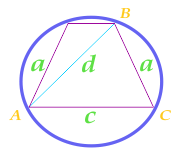To calculate the area of a circle, first calculate the semi-perimeter of a triangle ABC

a - side
c - side
d - diagonal
... preparation ...

18

Area of the circle described near the rectangle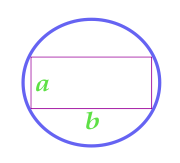a - side
b - side

... preparation ...

19

Area of a circle described near a regular polygon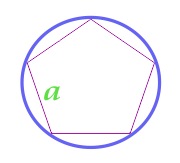a - side
N - the number of sides of the polygon

... preparation ...

20

Area of a circle described about a regular hexagon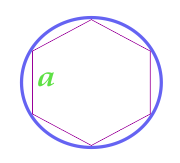a - side

... preparation ...